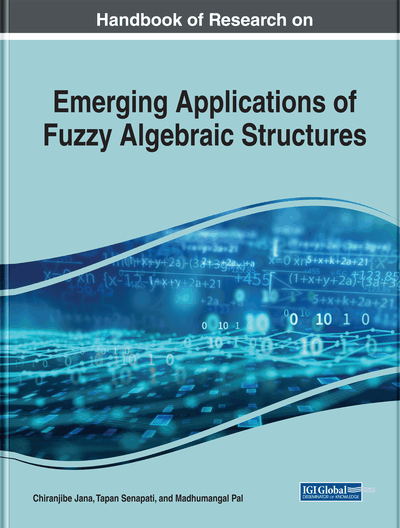Some Group Theoretic Notions in Fuzzy Multigroup Context

Paul Augustine Ejegwa (University of Agriculture, Nigeria)
DOI: 10.4018/978-1-7998-0190-0.ch003
Available
\$37.50
No Current Special Offers

Abstract

The notion of fuzzy multigroups is an application of fuzzy multisets or fuzzy bags to classical group theory. This chapter explores the notions of fuzzy comultisets, characteristic fuzzy submultigroups, and direct product of fuzzy multigroups as extensions of cosets, characteristic subgroups, and direct product of groups. The relationship between fuzzy comultisets of a fuzzy multigroup and the cosets of a group is established. Some results on the concept of fuzzy comultisets are deduced. A number of properties of characteristic fuzzy submultigroups of fuzzy multigroups are outlined, and some related results are obtained. Also, the author presents some properties of direct product of fuzzy multigroups and establish some results.
Chapter Preview
Top

1 Introduction

The concept of fuzzy sets was proposed by Zadeh (1965) as a method for representing imprecision in real-world situations. The concept of fuzzy set has grown stupendously over the years giving birth to fuzzy group proposed in (Rosenfeld, 1971). Some analogous concepts and results which were conventional in group theory have been established in fuzzy groups since inception. See (Ajmal and Prajapati, 1992; Bhattacharya and Mukherjee, 1987; Kim, 2003; Kumar, 1992; Mordeson et al., 2005; Mukherjee and Bhattacharya, 1984; Mukherjee and Bhattacharya, 1986; Onasanya and Ilori, 2013; Ray, 1999; Seselja and Tepavcevic, 1997; Sidky and Mishref, 1991) for details.

Motivated by Zadeh (1965), the idea of fuzzy multisets was introduced in (Yager, 1986) as a generalization of fuzzy sets in multisets framework. See (Biswas, 1999; Ejegwa, 2015; Miyamoto, 1996; Miyamoto and Mizutani, 2004; Mizutani et al., 2008; Syropoulos, 2012) for some details on fuzzy multisets. Recently, Shinoj et al. (2015) followed the foot steps of Rosenfeld (1971) and introduced a non-classical group called fuzzy multigroup. The concept of fuzzy multigroups constitutes an application of fuzzy multisets to the notion of group.

Baby et al. (2015) continued the algebraic study of fuzzy multisets by proposing the idea of abelian fuzzy multigroups. The work in (Ejegwa, 2018a), which was built on (Shinoj et al., 2015), introduced the concept of fuzzy multigroupoids and presented the idea of fuzzy submultigroups with a number of results. Some properties of abelian fuzzy multigroups were explicated in (Ejegwa, 2018b) with some results. The concept of normal fuzzy submultigroups of fuzzy multigroups was proposed in (Ejegwa, 2018c) with some number of results. In (Ejegwa, 2018d), the notion of homomorphism in the context of fuzzy multigroups was defined and some homomorphic properties of fuzzy multigroups were presented.

Since the concept of fuzzy multigroups is relatively noveltic, we seek to extend some notions hitherto established in group theory to fuzzy multigroups for the enrichment of fuzzy multigroup theory. Sequel, we explore the notions of fuzzy comultisets, characteristic fuzzy submultigroups and direct product of fuzzy multigroups. By organization, the chapter is thus presented: Section 2 provides some preliminaries on fuzzy multisets and fuzzy multigroups. In Section 3, we study the idea of fuzzy comultisets and discuss some of its properties. Section 4 discusses characteristic fuzzy submultigroups of fuzzy multigroups. In Section 5, we explicate the concept of direct product of fuzzy multigroups with some results. Finally, Section 6 concludes the paper and provides direction for future studies.

Top

2 Preliminaries

In this section, we review some existing definitions and results for the sake of completeness and reference.

Definition 2.1 Assume X is a set of elements. Then a fuzzy bag/multiset A drwan from X can be characterized by a count membership function CMA such that CMA: XQ, where Q is the set of all crisp bags or multisets from the unit interval I=[0,1].

A fuzzy multiset can also be characterized by a high-order function. In particular, a fuzzy multiset A can be characterized by a function CMA: XNI or CMA: X→[0,1]→N, where I=[0,1] andComplete Chapter List

Search this Book:
Reset